# Math in Focus Grade 3 Chapter 14 Practice 1 Answer Key Understanding Fractions

Practice the problems of Math in Focus Grade 3 Workbook Answer Key Chapter 14 Practice 1 Understanding Fractions to score better marks in the exam.

## Math in Focus Grade 3 Chapter 14 Practice 1 Answer Key Understanding Fractions

Find the fractions that make a whole.

Example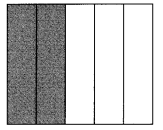$$\frac{2}{5}+\frac{3}{5}=\frac{5}{5}$$
= 1
Two-fifths and three-fifths make 1 whole.

Fill in the blanks.

Question 1.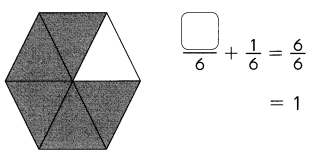___________ – ___________ and
___________ – ___________ make 1 whole.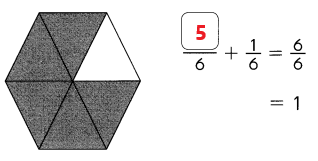Five – sixths and one – sixths make 1 whole.
Explanation:
In the above image we can observe five parts are shaded with black color out of six parts and one part is unshaded out of 6 parts. To make 1 whole we need to add 5/6 with 1/6.

Question 2.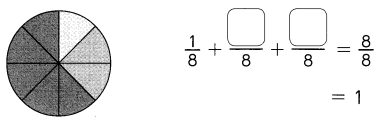___________ – ___________, ___________ – ___________, and ___________ – ___________ make 1 whole.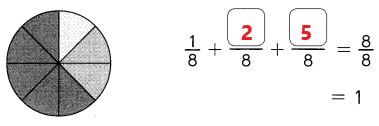one – eights, two eights and five eights make 1 whole.
Explanation:
In the above image we can observe 1 part is shaded with white color out of eight parts and two parts are shaded with gray color out of eight parts, five parts are shaded with gray color out of 8 parts. To make 1 whole we need to add 1/8, and 2/8 with 5/8.

Name the numerator and denominator.

Example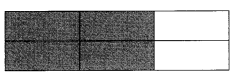$$\frac{4}{6}$$ of the figure is shaded.
In this fraction, the numerator is 4.
In this fraction, the denominator is 6.

Complete.

Question 3.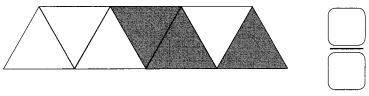of the figure is not shaded.
In this fraction, the numerator is ___________.
In this fraction, the denominator is ____________.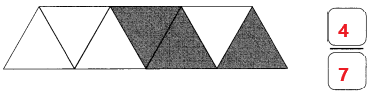of the figure is not shaded.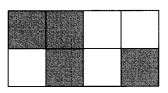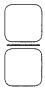of the figure is shaded.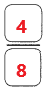of the figure is shaded.